# Periodic Classification of Elements Class 10 Extra Questions with Answers Science Chapter 5

In this page, you can find CBSE Class 10 Science Chapter 5 Extra Questions and Answers Periodic Classification of Elements Pdf free download, NCERT Extra Questions for Class 10 Science will make your practice complete.

## Class 10 Science Chapter 5 Extra Questions and Answers Periodic Classification of Elements

Extra Questions for Class 10 Science Chapter 5 Periodic Classification of Elements with Answers Solutions

### Periodic Classification of Elements Extra Questions Very Short Answer Type

Question 1.
What was Dobereiner’s basis of classifying elements?
According to Dobereiner, elements having similar properties can be arranged in triads in which atomic mass of the middle element is the mean of the atomic masses of the other two elements.Question 2.
State Mendeleev’s Periodic Law.
Mendeleev’s Periodic Law states that, “The properties of the elements are periodic function their atomic masses.”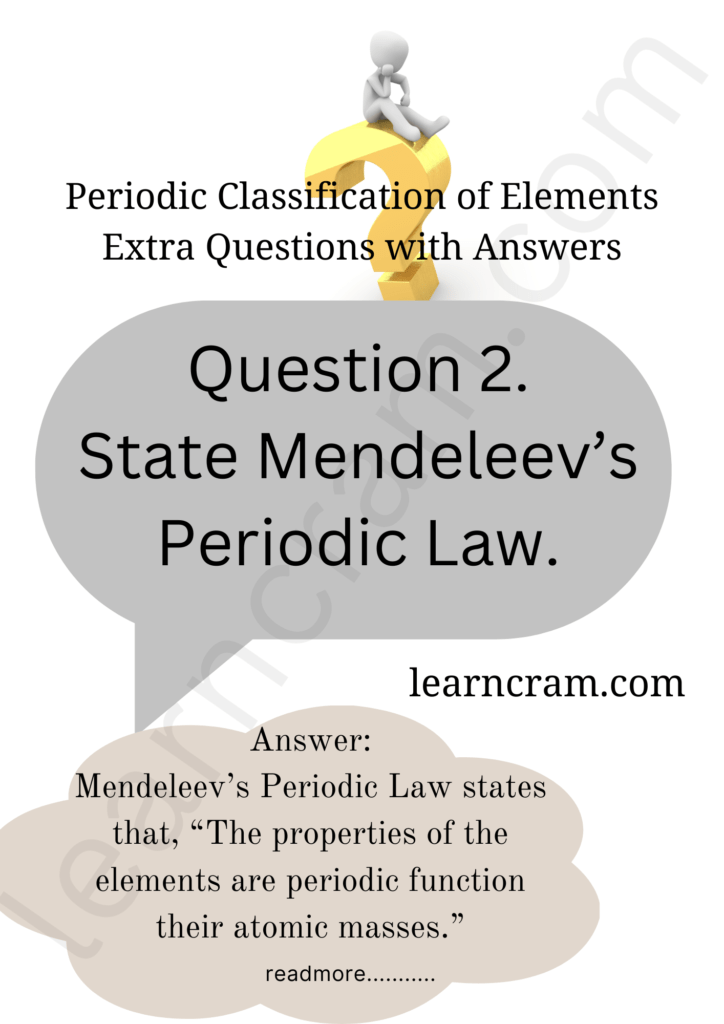Question 3.
State Newlands’ Law of Octaves.
Newland’s Law of Octave states that “when elements are arranged in order of increasing atomic weights, then every eighth element has properties similar to that of the first element.”

Question 4.
Who classified the elements on the basis of fundamental properties of elements like atomic mass.
Mendeleev.

Question 5.
Mendeleef’s periodic table was based on which law?
Mendeleef’s Periodic law.

Question 6.
What do you understand by periods in the Periodic Table?
The horizontal rows in the long form of the Priodic Table are called periods.

Question 7.
In how many groups and periods, the Modern Periodic Table of elements is divided?
18 groups and 7 priods.

Question 8.
Explain the diagonal relationship by taking exmaple of lithium (Li) and magnesium (Mg).
Diagonal Relationship : The first three members of second period show similarities with the members of their own groups and with the elements diagonally placed in the higher groups. This resamblance is called diagonal relationship.Question 9.
Write two main characteristics of Mendeleev’s periodic table and write name of elements of second period.
two main characteristics of Mendeleev’s periodic table are:
(i) It consists of 8 vertical columns called groups and 6 horizontal rows, called period.
(ii) In every period, elements are arranged in increasing order of their atomic masses.
Name of element of second period are lithium beryllium, boron, carbon, nitrogen, oxygen, fluorine.

Question 10.
Write four properties of modern periodic table.
Properties of modern periodic table are: –
(i) This table onsists of 18 vetical clumns called groups and 7 horizontal rows called periods.
(ii) Groups are not divided into subgroups.
(iii) Number of shells increases as we go down in a group.
(iv) Number of shells remains same in a period.

Question 11.
Give any two defect of Mendeleef’s periodic table.
(i) Position of Hydrogen : Position of Hydrogen was not fixed in the mendeleef’s periodic table.
(ii) Position of Isotopes : There is no place given isotopes in mendeleef’s periodic table.

### Periodic Classification of Elements Extra Questions Short Answer Type

Question 1.
The three elements A, B and C with similar properties have masses X, Y and Z respectively. The mass of Y is approximately equal to the average mass of X and Z. What is such an arrangement of elements called as? Give one example of such a set of elements.
The arrangement of these elements is known as Dobereiner triad.
Example: lithium, sodium and potassium.

Question 2.
Elements have been arranged in the following sequence on the basis of their increasing atomic masses.(a) Pick two sets of elements which have similar properties.
(b) The given sequence represents which law of classification of elements?
(a) (i) F and Cl, (ii) Na and K,
(b) Newland’s Law of Octaves.

Question 3.
Why did Mendeleev leave some gaps in his Periodic Table?
Mendeleev left some gaps in is Periodic Table for the elements yet to be discovered. He even predicted the properties of these elements by studying the properties of the neighbouring elements.

Question 4.
State Modern Periodic Law. What is number of groups and periods in the Modern Periodic Table?
Modern Periodic Law states that the properties of elements are a periodic function of their atomic number. There are 18 groups and 7 periods in the Modern Periodic Table.

Question 5.
What do you understand by the term periodicity? Does the periodicity in properties is a function of valence electrons? Illustrate.
When the elements are arranged in order of increasing atomic numbers, elements with similar chemical propertiers are repeated at definite intervals. This is known as periodicity. Yes, this periodicity is due to the periodicity in the number of electrons in the outermost shell of the atoms of the elements. If elements having the same number of valence electrons are grouped together, the elements falling within each group are similar in chemical properties.

Question 6.
What does each group in the Periodic Table signify?
Each group in the Periodic Table signifies identical outer shell electronic configuration for the elements.constituting that group. For example, each element of group I has one electron in its outermost shell.

Question 7.
Why do elements in any given group have similar properties?
The chemical properties of an atom are largely determined by its valence electrons. In a given group, the number of valence electrons are same, hence they have similar properties.

Question 8.
Why do group I elements form unipositive ions?
Group I elements contain 1 electron in their outermost shells. These elements lose this electron easily to attain the 8 electrons in their outermost shell. Hence, they form unipositive ion.Question 9.
Which is smaller?
(a) Na+ or Na
(b) Cl or Cl
(a) Na → 2,8,1 Na+ + 2,8
Na+ is smaller as it has lesser number of shells than Na.
(b) Cl → 2,8,7 Cl → 2,8,8
Cl has greater effective nuclear charge. So, it is smaller than Cl.

Question 10.
Why are the elements of group 18 called zero valent?
Group 18 elements have their outermost shells completely filled and the atoms of these elements have no tendency to gain or lose electrons. Thus, the elements of this group are zero valent and almost unreative.

Question 11.
Why group 17 elements form uninegative anions?
Group 17 elements have 7 valence electron less than the maximum number of electrons that can be accomodated in the outermost shell. Therefore, it is easier for the elements to gain an electron and form uninegative anions, so as to attain noble gas configuration. For example,Question 12.
Would you place the two isotopes of chlorine, Cl-35 and Cl-37 in different slots because their different atomic masses or in the same because their chemical properties are the same? Justify your answer.
In Mendeleev’s Periodic Table the two isotopes of chlorine, Cl-35 and Cl-37 would be placed in different slots because they have different atomic masses. But becauseof their similar chemical properties which is a more reliable property as it is based on the number of valence electrons, they would be placed in the same slot.

Question 13.
How does the metallic character change along the period?
As we go from left to right in a period, the metallic character decreases, because of the addition of an electron in the same energy shell each time. So, attraction between the nucleus and the electrons in the outermost shell increases. Thus, tendency to lose an electron decreases and metallic character decreases.

Question 14.
What is atomic radius? Why does atomic radius decrease across a period?
Atomic radius is defined as the distance from the centre of the nucleus of an atom to the outermost shell of electrons. The atomic radius decreases along a period. In moving from left to right across the period, the charge on the nucleus increases by one unit (due to increase in atomic number), but the additional electron goes to the same shell. As a result, outer electrons are pulled in closer to the nucleus. This causes the decrease of atomic size.

Question 15.
Why does the size of the atom increase down the group?
In moving down a group, the charge on the nucleus increases with increase in atomic number but at the same time, there is an increase in the energy levels. The number of electrons the outermost shell, however, remains the same. Since the effect of additional energy levels outweights the effect of increased nuclear charge and thus the distance of the outermost electron from the nucleus increases on going down the group.

Question 16.
Can the following groups of elements be classified as Dobereiner’s triad?
(a) Na, Si, ci
(b) Be, Mg, Ca
Atomic mass of Be 9; Na 23; Mg 24; Si 28; CI 35; Ca 40
Explain by giving reason.
(a) No, because all these elements do not have similar properties although the atomic mass of silicon is average of atomic masses of sodium (Na) and chlorine (Cl).
(b) Yes, because they have similar properties and the mass of magnesium (Mg) is roughly the average of the atomic masses of Be and Ca.

Question 17.
Write the formulae of chlorides of Eka-silicon and Eka-aluminium, the elements predicted by Mendeleev.
GeCl4, GaCl3.

Question 18.
If an element X is placed in group 14, what will be the formula and the nature of bonding of its chloride?
The formula will be XCl4. The element X of group 14 will have 4 valence electrons. So, it shares its 4 electrons and forms covalent bond with 4 chlorine atoms.

Question 19.
The atomic number of an element is 17. To which group and period does this element belong? Determine its valency.
Electronic configuration : 2, 8, 7;
Group: 17;
Period : 3;
Valency: 8 – 7 = 1

Question 20.
Arrange the following elements in increasing order of their atomic radii :
(a) Li, Be, F, N
(b) CI, At, Br, I
(a) F < N < Be < Li as the atomic radii decreases along a period.
(b) Cl < Br < I < At as the atomic radii increases down a group.

Question 21.
Identify and name the metals out of the following elements whose electronic configurations are given below:
(a) 2, 8, 2
(b) 2, 8, 1
(c) 2, 8, 7
(d) 2, 1
(a), (b) and (d) are metals.
(a) Magnesium (b) Sodium (d) Lithium.

Question 22.
Arrange the following elements in the increasing order of their metallic character
Mg, Ca, K, Ge, Ga
Ge < Ga < Mg < Ca < K.

Question 23.
Which group of elements could be placed in Mendeleev’s Periodic Table without disturbing the original order? Give reason.
Noble gases.
According to the Mendeleev’s classification, the properties of elements are the periodic function of their atomic masses and there is a periodic recurrence of elements with similar physical and chemical properties. Noble gases being inert, could be placed in a separate group without disturbing the original order.

Question 24.
(i) Why do we classify elements?
(ii) In Mendeleev’s Periodic Table, why was there no mention of noble gases like Helium, Neon and Argon?
(i) Different elements discovered in different times were found to posses different physical and chemical properties. But, it was difficult to study all the information or properties of these elements. Scientists started discovering some pattern in their properties to classify all the known elements in order to make their study easier. So, elements are classified on the basis of their similarities in properties.

(ii) Noble gases like He, Ne, Ar, etc. were not discovered at the time of Mendeleev. They were discovered later as they are inert and present in extremely low concentrations in our atmosphere. So, after their discovery, they were placed in a new group (18) in Modern Periodic Table.

Question 25.
Explain why sodium is more reactive than lithium.
Sodium is more reactive than lithium because sodium is larger in size. Outermost electrons are less tightly held in sodium than in lithium. As a result, sodium loses its outermost electron more easily than lithium. Hence, it is more reactive than lithium.

Question 26.
Choose from the following: 6C, 8O, 10Ne, 11Na, 14Si.
(i) Elements that should be in the same period.
(ii) Elements that should be in the same group.
(i) C, O, Ne should be in the same period as these elements contain same number of shells. Similarly, Na and Si should be in the same period.
(ii) C and Si should be in the same group as these elements have same number of valence electrons.

Question 27.
Write the main aim of classifying elements. Name the basic property of elements used in the development of Modern Periodic Table. State the Modern Periodic Law. On which side (part) of the Modern Periodic Table do you find metals, metalloids and non metals?
Elements are classified for systematic and simplified study of elements and their compounds. The basic property of elements used in the Modern Periodic Table is the atomic number of the elements.
Modern Periodic Law states that “the properties of elements are a periodic function of the atomic number.”
Metals are found on the left side and centre of the Modern Periodic Table.
Non-metals are found on the right side of the Modern Periodic Table.
Metalloids are found in a zig-zag manner between the metals and the non-metals.

Question 28.
How does the atomic radius of the elements change on going :
(i) from left to right in a period, and
(ii) down a group in the Modern Periodic Table? Give reason in support of your answer.
(i) Atomic radius decreases along a period. This is because nuclear charge increases from the right in a period which tends to pull the electrons closer to the nucleus.
(ii) Atomic radius increases down the group because number of shells increases on going down the group.

Question 29.
Name any two elements of group one and write their electronic configurations. What similarity do you observe in their electronic configuration? Write the formula of oxide of any of the aforesaid element.
Two elements of group I are Sodium (Na) and potassium (K).
Their electronic configurations : Na = 2, 8, 1; K = 2, 8, 8, 1
Similarity: Both have one valence electron.
Formula of their oxides : Na2O; K2O.

Question 30.
An element P (atomic number 20) reacts with an element Q (atomic number 17) to form a compound. Answer the following questions giving reason:
Write the position of P and Q in the Modern Periodic Table and the molecular formula of the compound formed when Preacts with Question
The electronic configuration of P is 2, 8, 8, 2.
Group – 2 because it has 2 valence electrons.
Period – 4 because it has 4 shells.
The electronic configuration of Q is 2, 8, 7.
Group – 17 because it has 7 valence electrons so group number is 7 + 10 i.e., 17.
Period – 3 because it has 3 shells.
Formula is PQ2 because valency of P is 2 and that of Q is 1.

Question 31.
Write the number of periods the Modern Periodic Table has. State the changes in valency and metallic character of elements as we move from left to right in a period. Also state the changes, if any, in the valency and atomic size of elements as we move down a group.
The Modern Periodic Table has 7 periods. Valency across a period increases from 1 to 4, the decreases from 4 to zero.
Metallic character of elements across a period decreases. Valency down a group remains the same. Atomic size of elements down a group increases.

Question 32.
The elements 4Be, 12Mg and 20Ca, each having two valence electrons in their valence shells are in periods 2, 3 and 4 respectively of the Modern Periodic Table. Answer the following questions associated with these elements, giving reason in each case :
(a) In which group should they be?
(b) Which one of them is least reactive?
(c) Which one of them has the largest atomic size?
(a) In group 2, as each element has two valence electrons.
(b) Be is least reactive because Be has the least tendency to lose electrons.
(c) Ca has the largest atomic size as Ca has the maximum number of shells and atomic size increases down the group.

Question 33.
Given below are some elements of the Modern Periodic Table. Atomic number of the element is given in the parentheses: A(4), B(9), C(14), D(19), E(20)
(a) Select the element that has one electron in the outermost shell. Also write the element configuration of this element.
(b) Which two elements amongst these belong to the same group? Give reason for your answer.
(c) Which two elements amongst these belong to the same period? Which one of the two has bigger atomic radius?
(a) D; the electronic configuration of D(19) is 2, 8, 8, 1.
(b) A and E belong to the same group as both have the same number of valence electrons, i.e.,
(c) A, B and D, E.
A has a bigger atomic radius than B and D has a bigger atomic radius than E.

Question 34.
Nitrogen (atomic no.7) and phosphorous (atomic no.15) belong to group 15 of the Periodic Table. Write the electronic configuration of these two elements in terms of K, L, M, N shell Predict whether these are metallic or non-metallic.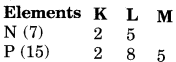Both are non-metals asthey tend to form bonds by gaining electrons.

Question 35.
Na, Mg and Al are the elements of the same period of Modern Table having one, two and three valence electrons respectively. Which of these elements
(i) has the largest atomic radius, (ii) is least reactive? Justify your answer stating reason for each case.
(i) Na has the largest atomic size because the atomic size decreases from left to right due to the increase in the nuclear charge.
(ii) Al is last reactive because the tendency to lose electrons decreases from left to right.

Question 36.
Two elements ‘P’ and ‘Q’ belong to the same period of the Modern Periodic Table and are in Group-1 and Group-2 respectively. Compare their following characteristics in tabular form:
(a) The number of electrons in their atoms
(b) The sizes of their atoms
(c) Their metallic characters
(d) Their tendencies to lose electrons
(e) The formula of their oxides
(f) The formula of their chlorides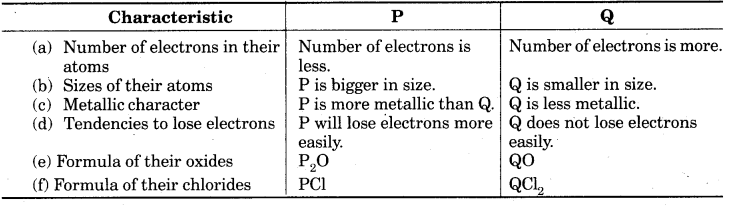Question 37.
The position of three elements A, B and C in the Periodic Table is shown below: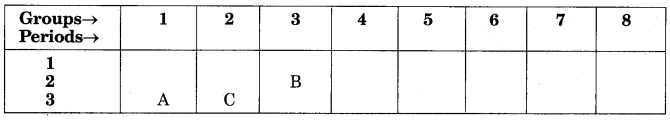(i) Element A belongs to group of the Periodic Table. So, it has 1 valence electrons and it forms unipositive ion by losing its valence electron. Hence, A is a metal.
(ii) This is because element C is in third period while element B is in second period. We know that the atomic size increases down the group and decreases along a period.
(iii) Element B is in 2nd period and 3rd group hence it has 3 valence electrons. It lose 3 electrons to achieve nearest inert gas configuration. So, its valency is 3.

Question 38.
Identify the elements with the following property and arrange them in increasing order of their reactivity.
(a) An element which is a soft and reactive metal.
(b) The metal which an important constituent of limestone.
(c) The metal which exists in liquid state at room temperature.
(a) Na or K (b) Ca (c) Hg
Reactivity order : Hg < Ca< Na < K

Question 39.
Properties of the elements are given below. Where would you locate the following elements of the Periodic Table?
(a) A soft metal stores under kerosene.
(b) An element with variable (more than one) valency stored underwater.
(c) An element which is tetravalent and forms the basis of organic chemistry.
(d) An element which is an inert gas with atomic number 2.
(e) An element whose thin oxide layer is used to make other elements corrosion resistance the process of “anodising”.
(a) Sodium (Na) Group 1 and Period 3 or Potassium (K) Group 1 and Period 4
(b) Phosphorus (P) Group 15 and Period 3
(c) Carbon (C) Group 14 and Period 2
(d) Helium (He) Group 18 and Period 1
(e) Aluminium (Al) Group 13 and Period 3.

Question 40.
The following table shows the position of six elements A, B, C, D, E and Fin the Periodic Table.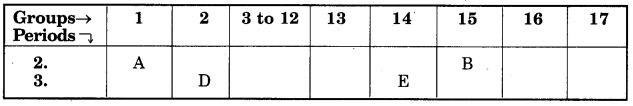Using the above table answer the following quesdtions:
(a) Which element will form only covalent compounds?
(b) Which element is a metal with valency?
(c) Which element is a non-metal with valency 3?
(d) Write a common name for the family of elements Cand E.
(e) Out of D and E, which one has a bigger atomic radius and why?
(a) E
(b) D
(c) B
(d) The noble gases.
(e) D has bigger atomic radius. The atomic radius decreases in moving from left to right along a period. This is due to an increase in nuclear charge which tends to pull the electrons close to the nucleus and reduces the size of the atom.

Question 41.
The atomic number of an element ‘X’ in the periodic table.
(ii) Write the formula of the compound formed when ‘X’ reacts/combines with another element ‘Y’ (atomic number 8).
(iii) What would be the nature (acidic or basic) of the compound formed? Justify the answer.
Electronic configuration : 2, 8, 8, 2
(i) ‘X’ is in the 2nd group and 4th period of the periodic table.
(ii)(iii) The nature of the compound formed is basic because X is a metal and Y is oxygen. The oxides of metals are basic in nature.

Question 42.
The atomic radii of first group elements of the Periodic Table are as follows:(i) Arrange these elements in the increasing order of their atomic radii.
(ii) Name the elements which have the smallest and the largest atoms.
(iii) How does the atomic size vary as you go down a group?
(i)(ii) The element Li has the smallest atom (atomic radius 152 pm) whereas the element Cs has the largest atom (atomic radius 262 pm).
(iii) From this arrangement, we find that the atomic size (or radius) increases down the group.

Question 43.
The elements of the second period of the Periodic Table are givne below:(i) Explain why atomic radius decreases from Li to F.
(ii) Identify the most metallic and non-metallic elements among the above elements.
(i) On moving from left to right along a period of the Periodic Table, atomic number increases, i.e., number of protons and electrons increases which ultimately increases the nuclear charge. Hence, the electrons are pulled in closer to the nucleus which leads to contraction of the atom and thus atomic radius decreases.

(ii) On moving from left to right in a Periodic Table, metallic character decreases and non metallic character increases. This is because the capacity to lose electrons from the outermost orbit of an atom (called ionisation energy) decreases. Hence, among the above element Li being placed on the extreme left side is most metallic.and F being placed on the extreme right side is the most non-metallic element.

Question 44.
The atomic number of nitrogen, oxygen and fluorine are 7, 8 and 9 respectively. Write the electronic configuration of each element and answer the following questions:
(a) Which one of N, O and F is most electronegative and which on is least electronegative?
(b) What is the number of valence elecrtons of F?
(c) What is valency of each one of N, O and F?
Electronic configuration of:
Nitrogen : 2, 5
Oxygen : 2, 6
Fluorine : 2, 7
(a) Fluorine (F) is the most electronegative and nitrogen (N) is the least electronegative.
(b) Fluorine (F) has 7 valence electrons.
(c) Valency of nitrogen is 3 and 5, valency of oxygen is 2, valency of fluorine is 1.

Question 45.
Four elements P, Q, R and S belong to the third period of the Modern Periodic Table and have respectively 1, 3, 5 and 7 electrons in their outermost shells. Write the electronic configurations of Q and Rand determine their valencies. Write the molecular formula of the compound formed when P and S combine.
Electronic configuration of Q : 2, 8, 3
Valency of Q : 3
Electronice configuration of R : 2, 8, 5
Valency of R : 8 – 5 = 3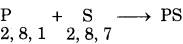Question 46.
Consider the following elements :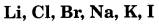(i) Arrange the elements according to the groups to which they belong in the Periodic Table.
(ii) What are the common properties on the basis of which the elements have been grouped together?
(i) Li, Na and K are grouped together in the periodic table and belong to group I. They are grouped together because all of them have one electron in their valence shell. Rest of the elements Cl, Br and I are grouped together in the periodic table and belong to group 17.
(ii) The elements of group I are called alkali metals because they react with water to liberate H, gas and form alkalis. The elements of group 17 are monovalent non-metals. They form acidic oxides and are called halogens.

Question 47.
Write the names given to the vertical columns and horizontal rows in the Modern Periodic Table. How does the metallic character of elements vary on moving down a vertical column? How doesd the size of ayomic radius vary on moving left to right in a horizontal row? Give reason in support of your answer in the above two cases.
Vertical columns – Groups
Horizontal rows – Period
Metallic character increases on moving down a vertical column. This is because ability to lose electrons increases on moving down the group due to increase in distance between the nucleus and the valence electrons.

Atomic radius decreases on moving left to right across a period because the nuclear charge increasves on moving from left to right across a period resulting in increase in the attraction between the nucleus and the valence electrons.

Question 48.
Atomic number is considered to be more appropriate parameter than atomic mass for classification of elements in a periodic table. Why? How does metallic character of elements vary on moving from:
(i) left to right in a period?
(ii) from top to bottom in a group? Give reasons for your answers.
The properties of elements depend upon valence electrons in the atom which in turn depends on the total number of electrons, i.e., atomic number. Therefore, atomic number is a more appropriate parameter than atomic mass for classification of elements.
(i) On moving from left to right in a period, the metallic character decreases. This is due to an increase in nuclear charge which tends to pull the electrons closer to the nucleus and reduces the size of the atom.
(ii) On moving from top to bottom in a group, metallic character increases. This is because new sells are being added as we go down the group. This increases the distance between the valence shell and the nucleus.

Question 49.
Two elements with symbol X (atomic no. 16) and Y (atomic no.12) are placed in the III period of the Modern Periodic Table. (i) Which amongst the two has more metallic character?
(ii) Calculate the valency of each element.
(iii) Element ‘Y’ is smaller than ‘X’ in terms of atomic size. Is the statement true, justify?
(i) Y
(ii) 2 each
(iii) False, same period but Y lies in group 2 and X in group 16. So, as we move from left to right size decreases with increase in nuclear charge.

Question 50.
Taking the example of an element of atomic number 16, explain how the electronic configuration of the atom of an element relates to its position in the Modern Periodic Table and how valency of an element is calcualted on the basis of its atomic number.
Atomic number of the element = 16
Electronic configuration = 2, 8, 6
Period number = Number of shells The atom of the element has three shells. So, the period number is 3.
The atom of the element has six valence electrons. So, the group number of the element will be 16(6 + 10).
Valency = 8 – number of valence electrons (> 5) or number of valence electrons (< 5).

Question 51.
Calcium is an element with atomic number 20. Stating reason answer each of the following questions:
(i) Is calcium a metal or non-metal?
(ii) Will its atomic radius be larger or smaller than that of potassium with atomic number 19?
(iii) Write the formula of its oxide.
(i) Calcium is a metal sicne it has two electrons in its outermost shell which it can lose easily.
(ii) K(19) is placed before Ca(20) in the same period (fourth period). Since the atomic radius decreases along a period, the atomic radius of calcium is smaller than that of potassium.
(iii) The formula of oxide of calcium is CaO, because the valency of calcium as well as that of oxygen is 2.

### Periodic Classification of Elements Extra Questions Long Answer Type

Question 1.
(a) How do you calculate the valency of an element from its electronic configuration?
(b) What is the valency of magnesium with atomic number 12 and sulphur with atomic number 16?
(c) How does the valency vary in a period on going from left to right?
(d) How does the valency vary in going down a group?
(a) The valency of an element is determined by the number of valence electrons present in the outermost shell of its atom. The number of electrons lost or gained (or shared) by one atom of an element to achieve the nearest inert gas electron configuration, gives us the valency of the element.

(b) (i) Atomic number of magnesium = 12
Electronic configuration is 2, 8, 2.
A magnesium atom can lose its 2 valence electrons to achieve the inert gas electronic configuration of neon (2,8).
Therefore, valency of magnesium = 2.

(ii) The atomic number of sulphur = 16.
Electronic configuration = 2, 8, 6.
A sulphur atom cannot lose 6 electrons to achieve inert gas electronic configuration to energy consideration. It can gain 2 electrons to achieve the nearest inert gas electronic configuration of argon (2, 8, 8).
Thus, the valency of sulphur = 2.

(c) On going from left to right along a period (short period), the valency of elements increase from 1 to 4 and then decreases to 0 (zero).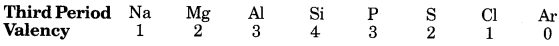(d) All the elements in a group have the same valency.

Question 2.
An element placed in 2nd Group and 3rd Period of the Periodic Table, burns in presence of oxygen to form a basicoxide.
(a) Identify the element.
(b) Write the electronic configuration.
(c) Write a balanced equation when it burns in the presence of air.
(d) Write a balanced equation when this oxide is dissolved in water.
(a) Magnesium (Mg)
(b)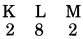(c) 2Mg(s) + O2 (g) → 2MgO (s)
(d) MgO(s) + H2O (l) → 2Mg(OH)2 (aq)

Question 3.
Atomic number of a few elements are given below:
10, 20, 7, 14
(a) Identify the elements.
(b) Identify the Group number of these elements in the Periodic Table.
(c) Identify the Periods of these elements in the Periodic Table.
(d) What would be the electronic configuration for each of these elements?
(e) Determine the valency of these elements.
(a) Elements – Neon (Ne), Calcium (Ca), Nitrogen (N), Silicon (Si)
(b) Group-18, 2, 15, 14
(c) Period – 2, 4, 2, 3
(d) Electronic configuration – (2, 8); (2, 8, 8, 2); (2, 5); (2, 8, 4)
(e) Valency – 0, 2, 3, 4

Question 4.
Mendeleev predicted the existence of certain elements not known at that time and named two of them as Eka-silicon and Eka-aluminium.
(a) Name the elements which have taken the place of these elements.
(b) Mention the Group and the Period of these elements in the Modern Periodic Table.
(c) Classify these elements as metals, non-metals or metalloids.
(d) How many valence electrons are present in each one of them?
(a) Germanium (Ge) and Gallium (Ga).
(b) Group 14; Period 4 and Group 13; Period 4
(c) Ge–Metalloid; Ga–Metal
(d) Ga–3; Ge 4

Question 5.
An element X which is a yellow solid at room temperature shows catenation and allotropy.X forms two oxides which are also formed during the thermal decomposition of ferrous sulphate crystals and are the major air pollutants.
(a) Identify the element X.
(b) Write the electronic configuration of X.
(c) Write the balanced chemical equation for the thermal decomposition of ferrous sulphate crystals.
(d) What would be the nature (acidic/basic) of oxides formed?
(e) Locate the position of the element in the Modern Periodic Table.
(a) Element X is sulphur (atomic no. 16)
(b)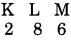(c)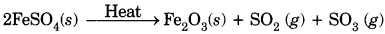(d) Acidic
(e) 3rd period, group 16.

Question 6.
Give an account of the process adopted by Mendeleev for the classification of elements. How did he arrive at “Periodic Law”?
(i) When Mendeleev started his work, 63 elements were known. He studied the compounds of these elements with oxygen and hydrogen. He selected hydrogen and oxygen as they are very reactive and formed compounds with most elements. The formulae of the hydrides and oxides formed by an element were treated as one of the basic propeties of an element for its classification.
(ii) Elements with similar properties were arranged in a group.
(iii) Mendeleev observed the elements were automatically arranged in the order of increasing atomic masses.

Question 7.
The atomic number of an element ‘X’ is 19.
(a) Write its electronic configuration.
(b) To which period of the Modern Periodic Table does it belong and what is its valency?
(c) If X’ burns in oxygen to form its oxide, what will be its nature-acidic, basic or neutral?
(d) Write balanced chemical equation for the reaction when this oxide is dissolved in water.
(a) Configuration of X (19) = 2, 8, 8, 1.
(b) It belongs to fourth period and its valency is 1.
(c) Basic oxide (X2O)
(d) X2O + H2O → 2XOH

Question 8.
(a) How does the atomic radius change as you go :
(i) from left to right in a period?
(ii) down a group in the periodic table?
(b) Two elements X and Y have atomic numbers 12 and 16 respectively. Write the electronic configuration for the these elements. To which period of the Modern Periodic Table do these two elements belong? What type of bond will be formed between them and why?
(a) (i) Atomic radius of decreases.
(b) Atomic number of element X = 12The period number of an element is equal to the number of electron shells in its atom. These two elements have 3 electron shells, therefore they belong to 3rd period.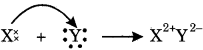They will form ionic bond because X is a metal and Y is a non-metal. X loses two electrons which will be gained by Y.

Question 9.
(a) How would the tendency to lose electrons change as you go :
(i) from left to right across a period?
(ii) down a group?
(b) An element X (2, 8, 2) combines separately with (NO3), (SO4)2- and (PO4)3- radicals. Write the formulae of the three compounds so formed. To which group of the periodic table does the element ‘X’ belong? Will it form covalent or ionic compound? Why?
(a) (i) Tendency to lose electrons decreases.
(ii) Increases.
(b) The three compounds are X (NO3)2, XSO4, and X3(PO4)2.
Valence electrons of element X is 2. Therefore, element X belongs to group 2. It will form ionic compounds because X loses 2 electrons to achieve the electronic configuration of inert gas and forms a positively charged ion.

Question 10.
The position of eight elements in the Modern Periodic Table is given below where atomic numbers of elements are given in the parenthesis.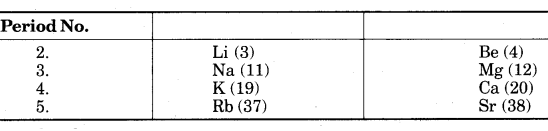(i) Write the electronic configuration of Ca.
(ii) Predict the number of valence electrons in Rb.
(iii) What is the number of shells in Sr?
(iv) Predict whether K is a metal or a non-metal.
(v) Which one of these elements has the largest atom in size?
(vi) Arrange Be, Ca, Mg and Rb in the increasing order of the size of their respective atoms.
(i) Electronic configuration of Ca -2, 8, 8, 2
(ii) Valence electrons in Rb is 1.
(iii) Five
(iv) Metal
(v) Rb is biggest in size.
(vi) Be < Mg < Ca < Rb

Question 11.
Atoms of eight elements A, B, C, D, E, F, G and H have the same number of electronic shells but different number of electrons in their outermost shell. It was found that elements A and G combine to form an ionic compound which can also be extracted from sea water. This compound is added in a small amount to almost all vegetable dishes during cooking. Oxides of elements A and B are basic in nature while those of E and F are acidic. The oxide of D is almost neutral. Based on the above information, answer the following questions :
(i) To which group or period of the periodic table do the listed elements belong?
(ii) What would be the nature of compounds formed by a combination of elements B and F?
(iii) Which two of these elements could definitely be metals and which are likely to be non-metals?
(iv) Which one of the eight elements is most likely to be found in gaseous state at room temperature?
(v) If the number of electrons in the outermost shell of elements C and G be 3 and 7 respectively, write the formula of the compound formed by the combination of C and G.
(vi) Which one of the eight elements is likely to be a noble gas?
(vii) Which one of the eight elements would have the largest atomic radius?
(viii) Which one of these eight elements is likely to be a semi-metal or metalloid?
(i) Third period
Group—1, 2, 13, 14, 15, 16, 17, 18 respectively.
(ii) Nature of compound : Electrovalent/ionic
(iii) Metals—A and B ; Non-metals—E, F, G
(iv) G/H
(v) CG3
(vi) H
(vii) A
(viii) D

Question 12.
Atoms of seven elements A, B, C, D, E, F and G have a different number of electronic shells but have the same number of electrons in their outermost shells. The elements A and C combine with chlorine to form an acid and common salt respectively.. The oxide of element A is liquid at room temperature and is a neutral substance, while the oxides of the remaining six elements are basic in nature. Based on the above information answr the following questions :
(i) What could the element A be?
(ii) Will element A to G belong to the same period or same group of the periodic table?
(iii) Write the formula of the compound formed by the reaction of the element A with oxygen.
(iv) Show the formation of the compound by a combination of element C with chlorine with the help of electronic structure.
(v) What would be the ratio of numbr of combining atoms in a compound formed by the combination of element A with carbon?
(vi) Which one of the given elements is likely to have the smallest atomic radius?
(i) Hydrogen
(ii) Same group
(iii) A2O/H2O
(iv)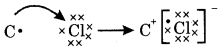(v) 4:1
(vi) A

Question 13.
In the following table, six elements A, B, C, D, E and F of the modern periodic table with atomic numbers 3 to 18 are given :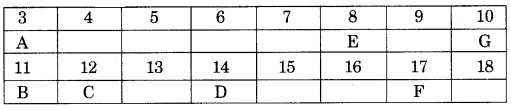(a) Which of these is (i) Noble gas, (ii) halogen?
(b) Which of these is the most active metal in 3rd period?
(c) Identify the most electronegative element in the third period.
(d) In the compound between B and F what type of bond wiīl be formed?
(e) What would be the nature of oxide formed by C?